# The PHREG Procedure

### Using the TEST Statement to Test Linear Hypotheses

Linear hypotheses for
are expressed in matrix form as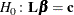where L is a matrix of coefficients for the linear hypotheses, and c is a vector of constants. The Wald chi-square statistic for testing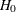is computed as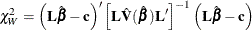where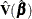is the estimated covariance matrix. Under,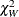has an asymptotic chi-square distribution with r degrees of freedom, where r is the rank of.

#### Optimal Weights for the AVERAGE option in the TEST Statement

Let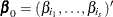, where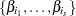is a subset of s regression coefficients. For any vector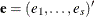of length s,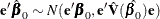To findsuch that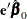has the minimum variance, it is necessary to minimize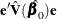subject to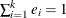. Let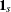be a vector of 1’s of length s. The expression to be minimized is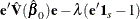where
is the Lagrange multiplier. Differentiating with respect toand, respectively, yields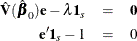Solving these equations gives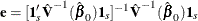This provides a one degree-of-freedom test for testing the null hypothesis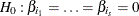with normal test statistic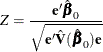This test is more sensitive than the multivariate test specified by the TEST statement

Multivariate: test X1, ..., Xs;


where X1, …, Xs are the variables with regression coefficients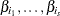, respectively.